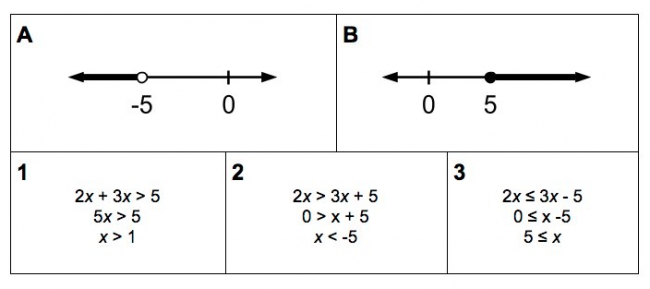# Representing Solutions to Inequalities

Preview:This task is intended for students to match worked examples of inequalities to how the solutions to those inequalities are represented on a number line.

Connecting Representations
Content:
solving inequalities, representing the solutions to inequalities
Curricular Competency:
Reasoning and analyzing: Develop mental math strategies and abilities to make sense of quantities
Understanding and solving: Develop, demonstrate, and apply mathematical understanding through play, inquiry, and problem solving
Understanding and solving: Visualize to explore mathematical concepts
Understanding and solving: Develop and use multiple strategies to engage in problem solving
Communicating and representing: Communicate mathematical thinking in many ways
Communicating and representing: Use mathematical vocabulary and language to contribute to mathematical discussions
Communicating and representing: Explain and justify mathematical ideas and decisions
Communicating and representing: Represent mathematical ideas in concrete, pictorial, and symbolic forms
Connecting and reflecting: Reflect on mathematical thinking